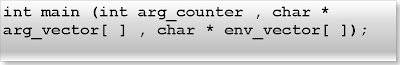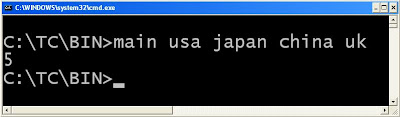## INDEX

### main.c

Every c program starts with a main function and end with null statement.

Properties of main function:

1. Any c program can have only one main function.

2. main function is called by operating system.

3. main is not keyword of c. So any variable name can be main.

4. Programmer can also call the main function.

5. Default return type of main function is int and default parameter is void.

Prototype of main function in c:A main function in c has three parameters. They are:

1. Argument counter

2. Argument vector

3. Environment vector

1. Argument counter: First parameter of main function is argument counter. It stores an integer number which is equal to number of parameters passed including file name from the command prompt.

Example:

Step1: Write the following code in your c compiler.

int main(int arg_counter){

printf("%d",arg_counter);

return 0;

}

Step2: Save the file (Let main.c) and compile the program

Step 3: Open command prompt go to the directory where you have save your file (i.e. main.c)

Step 4: Write following in the command prompt:

main usa japan china uk (Press enter key)You will get 5 as an output because we have passed five parameters including file name (i.e. main).

2. Argument vector: Second parameter of main function is argument vector. It is array which is array of string. This string array contains the actual parameters which have passed from the command prompt.

Example:

//main.c

void main(int count ,char *arg_vector[]){

int i;

for(i=0;i

printf("%s\n",arg_vector[i]);

}

}

In the command prompt write:

main usa japan china uk (Press enter key)

Output:

main

usa

japan

china

uk

3. Environment vector

Third parameter of main function is environment variable. It is also array of string which contains all the environments variables of the system.

Example:

void main(int count ,char *arg_vector[],char *env_vector[]){

int i;

for(i=0;i

printf("%s\n",env_vector[i]);

}

}

Conceptual questions on main function:

(1) What will be output if you will execute following c code?

#include "process.h"

int main(){

static int x=5;

if(x<10){

printf("\nHello");

x++;

main();

}

else {

getch();

exit(0);

}

return 0;

}

Output:

Hello

Hello

Hello

Hello

Hello

Explanation: Programmer can call the main function.

(2) What will be output if you will execute following c code?

void main(){

int main=10;

main++;

printf("%d",main);

getch();

}

Output:

11

Explanation: main is not keyword of c. A variable name can be main but it is bad practice.

(3) What will be output if you will execute following c code?

void one();

void two();

#pragma startup one

#pragma exit two

void main(){

printf("main");

}

void one(){

printf("one");

}

void two(){

printf("two");

getch();

}

Output:

One

main

two

Explanation: Every c program starts from main function and end will null statement. But with the help of preprocessor directive it is possible to call any function just before staring of function and call the function just after the ending of function.

(4) What will be output if you will execute following c code?

#include "stdio.h"

void main(){

clrscr();

printf("main");

getch();

return;

printf("go");

getch();

}

Output:

main

Explanation: A c program will terminate when control encounters any null statement.